Core Java - Interview Questions and Answers for 'Interesting questions' | Search Interview Question - javasearch.buggybread.com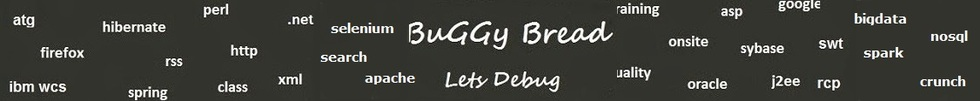# Search Interview QuestionsMore than 3000 questions in repository.There are more than 900 unanswered questions.Have a video suggestion.
Click Correct / Improve and please let us know.
Label / Company      Label / Company / TextSubmit Question

Core Java - Interview Questions and Answers for 'Interesting questions' - 5 question(s) found - Order By NewestQ1. How to find whether a given integer is odd or even without use of modulus operator in java? Core Java
Ans. public static void main(String ar[])
{
int n=5;
if((n/2)*2==n)
{
System.out.println("Even Number ");
}
else
{
System.out.println("Odd Number ");
}
}Help us improve. Please let us know the company, where you were asked this question :LikeDiscussCorrect / Improvejava   code   coding   tricky questions   interesting questions   modulus operator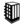Asked in 4 Companies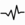frequentQ2. What will be the output of following Code ? class BuggyBread {    public static void main(String[] args) {       String s2 = "I am unique!";       String s5 = "I am unique!";       System.out.println(s2 == s5);    } } Core Java
Ans. true, due to String Pool, both will point to a same String object.Help us improve. Please let us know the company, where you were asked this question :LikeDiscussCorrect / Improvejava   code   coding   tricky questions   interesting questions   string   string pool   .equal   ==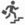intermediatefrequentQ3. What will be the output of following code ? class BuggyBread2 {           private static int counter = 0;         void BuggyBread2() {               counter = 5;        }             BuggyBread2(int x){       counter = x;        }            public static void main(String[] args) {               BuggyBread2 bg = new BuggyBread2();               System.out.println(counter);        }  } Core Java
Ans.  Compile time error as it won't find the constructor matching BuggyBread2().
Compiler won't provide default no argument constructor as programmer has already defined one constructor.
Compiler will treat user defined BuggyBread2() as a method, as return type ( void ) has been specified for that.Help us improve. Please let us know the company, where you were asked this question :LikeDiscussCorrect / Improvejava   code   coding   tricky questions   interesting questions   default constructor   constructor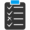Try 3 Question(s) TestQ4. If you have access to a function that returns a random integer from one to five, write another function which returns a random integer from one to seven. Core Java
Ans. We can do that by pulling binary representation using 3 bits ( random(2) ).

getRandom7() {
String binaryStr = String.valuesOf(random(2))+String.valuesOf(random(2))+String.valuesOf(random(2));
binaryInt = Integer.valueOf(binaryStr);
int sumValue=0;
int multiple = 1;
while(binaryInt > 0){
binaryDigit = binaryInt%10;
binaryInt = binaryInt /10;
sumValue = sumValue + (binaryDigit * multiple);
multiple = multiple * 2;
}
}Help us improve. Please let us know the company, where you were asked this question :LikeDiscussCorrect / Improvejava   tricky question   interesting questions   google interview questions   randomQ5. Write a method to convert binary to a number ? Core Java
Ans.
convert(int binaryInt) {
int sumValue=0;
int multiple = 1;
while(binaryInt > 0){
binaryDigit = binaryInt%10;
binaryInt = binaryInt /10;
sumValue = sumValue + (binaryDigit * multiple);
multiple = multiple * 2;
}
return sumValue;
}Help us improve. Please let us know the company, where you were asked this question :LikeDiscussCorrect / Improvejava   binary   interesting question   coding

## Help us and Others Improve. Please let us know the questions asked in any of your previous interview.

Any input from you will be highly appreciated and It will unlock the application for 10 more requests.